Technical Article

# Learn by Example—Using an Impedance Smith Chart

March 17, 2023 by Dr. Steve Arar

## Learn how a series RLC circuit with arbitrary component values can be represented as a point on the Smith chart and how an impedance contour on the Smith chart can be used to describe the circuit's frequency response.

The Smith chart is a powerful graphical tool that is extensively used by RF engineers to rapidly determine how a given impedance can be transformed into another one. Since RF circuit simulators and measurement equipment use the Smith chart as a standard way of presenting their output, it’s important to be familiar enough with it to easily recognize the impedance and Γ values associated with different parts of the chart. The Smith chart was an invention of the electrical engineer Phillip Hagar Smith.

In this article, we’ll get more familiar with the impedance Smith chart. We’ll discuss that a series RLC circuit with arbitrary component values can be represented as a point on the Smith chart. We’ll also see that an impedance contour on the Smith chart can be used to describe the frequency response of the circuit.

### The Smith Chart Mapping Function: Key Points

To more easily recognize the correspondence between different z and Γ values on a Smith chart, it is helpful to remember the mapping function that produces the Smith chart. This mapping function is repeated below from a previous article for convenience:

$\Gamma = \frac{z-1}{z+1}$

##### Equation 1.

Figure 1 shows a Smith chart with some constant-resistance and constant-reactance contours obtained using the above mapping function.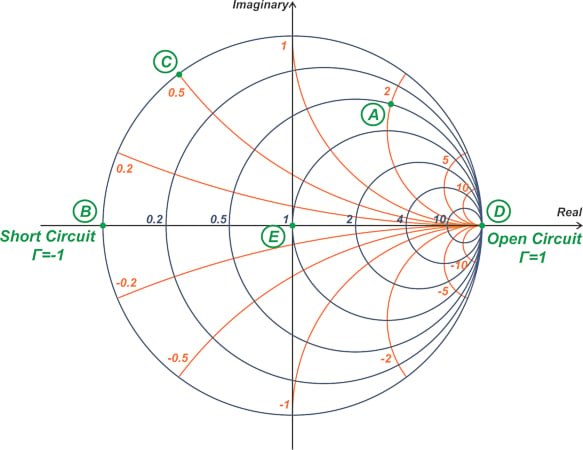##### Figure 1. Example Smith chart with some constant-resistance and constant-reactance contours.

To locate a normalized impedance of z = r + jx, we find the intersection of the corresponding constant-resistance circle and constant-reactance arc. For example, the impedance z = 0.5 + j2 is marked as point A in the above figure. In many cases, the actual circles and arcs that you need are not present in your Smith chart, and you have to use the available circles and arcs to estimate the impedance location.

For a short circuit, we substitute z = 0 into Equation 1 and obtain Γ = -1, marked as point B in Figure 1. On the other hand, for an open circuit (z = ∞), Γ works out to +1, denoted as point D in the figure.

Additionally, the outermost circle on the Smith chart is the circle of unit radius in the Γ-plane and corresponds to impedances with r = 0. At point B, x is also 0, and we have a short. At point D, x tends to infinity, and we have an open circuit.

For any impedance with r = 0, we’re on the unit circle; therefore, for r = 0, the magnitude of Γ is unity, and its phase angle changes depending on the x component of z. For example, z = j0.5 gives us point C in Figure 1. Increasing x from 0 to +∞ will take us from point B to D along the upper half of the unit-radius circle. If we sweep x from 0 to -∞, we’ll again end up at point D; however, this time, through the lower half of the unit circle.

Note that other constant-resistance circles also pass through point D (the Γ = 1 point). This makes sense because no matter what the value of r is, you’ll get an open circuit if you keep increasing the magnitude of x to larger and larger values.

The horizontal diagonal of the unit circle (the real axis in the figure) corresponds to purely resistive impedances (impedances of the form z = r + j0). For example, with a resistor equal to the normalizing impedance z = 1, we’re at the center of the Smith chart (point E) where Γ = 0. Therefore, the center of the Smith chart corresponds to a load with zero reflection coefficient.

The arcs in the upper half of the Smith chart are contours of constant reactance impedances with positive x. Thus, the inductive impedances appear in the upper half of the chart. On the other hand, the lower half, which includes the negative values of x, corresponds to capacitive impedances. Another helpful observation is that smaller circles and arcs correspond to larger resistance and reactance values, respectively. Meaning that if you increase the r or x component of the impedance, you’ll move toward smaller circles and arcs in the Smith chart.

A series RLC circuit with arbitrary component values can be represented by an equivalent normalized impedance r + jx at a given frequency, and thus a point on the Smith chart can be easily used to represent the impedance of a series RLC circuit. If we sweep the frequency, the imaginary part of the impedance changes. In this case, an impedance contour can be plotted on the Smith chart to describe the circuit behavior over the swept frequency range. Let’s look at some examples.

#### Example 1: Adding a Series Capacitor

With a normalized load impedance of z= 0.5 + j, the reflection coefficient is Γ= 0.62 $$\angle$$ 82.87° (Equation 1). If we add to this impedance a 10 pF series capacitor (C= 10 pF), what would be the new impedance and reflection coefficient? Assume that the operating frequency is 211.7 MHz and the reference impedance is Z= 50 Ω.

The load impedance z1 and the associated reflection coefficient Γ1 are shown in Figure 2.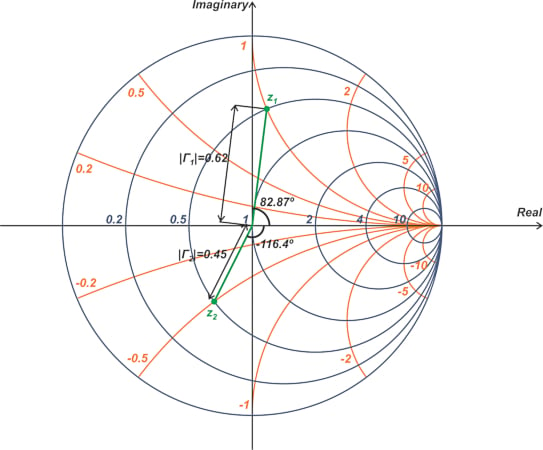##### Figure 2. Smith chart showing the load impedance z1 and the associated reflection coefficient Γ1.

Since z1 has an inductive reactance, it appears at the upper half of the Smith chart.

At 211.7 MHz, a 10 pF capacitor has a normalized reactance of -j1.5:

$\begin{eqnarray} jx &=& -\frac{j}{2 \pi f CZ_0} \\ &=& -\frac{j}{2 \pi \times 211.7 \times 10^6 \times 10 \times 10^{-12} \times 50}=-1.5j \end{eqnarray}$

The series addition of the capacitor changes only the reactive component of z1. This means that the new impedance also lies on the r = 0.5 constant-resistance circle. However, the capacitor reduces the initial reactance by j1.5, moving us from the j1 to -j0.5 constant-reactance arc. Therefore, as shown in Figure 2, the new impedance z2 is at the intersection of the r = 0.5 constant-resistance circle and the x = -0.5 constant-reactance arc. Measuring the length and phase angle of the vector from the origin to z2, we obtain Γ= 0.45 $$\angle$$ -116.4°.

Now assume that we add a second series 10 pF capacitor (C= 10 pF) to z2, what would be the new impedance z3? The new capacitor reduces the reactance by another j1.5. Therefore, we end up at point z= 0.5 - j2, as shown below (Figure 3).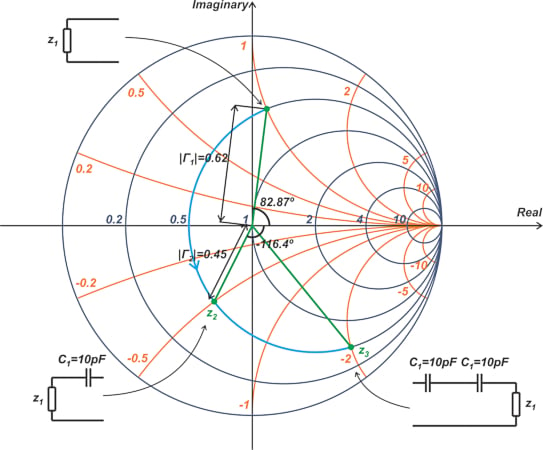##### Figure 3. Smith chart showing point z3.

The above example shows that by continuing to add more and more series capacitors, the overall impedance moves along the constant-resistance circle in a counterclockwise direction.

#### Example 2: Sweeping the Value of a Series Capacitor

If we add a series capacitor Cs to a normalized load impedance of z= 0.5 + j and sweep the capacitor value from +∞ to 5 pF, what would be the overall impedance contour on the Smith chart?

We can use the results of the previous example to answer this one. To this end, we replace the circuit schematics of Figure 3 with their equivalent circuits, as shown below in Figure 4.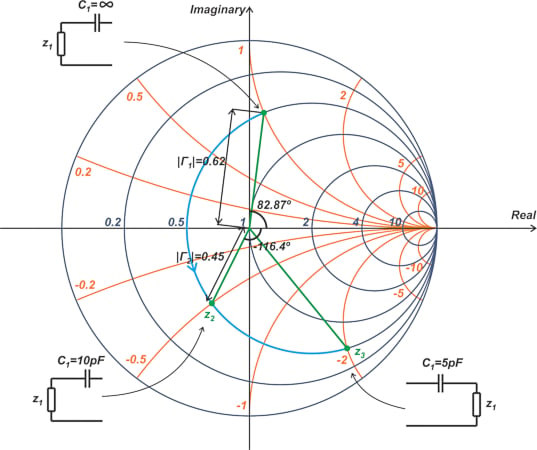##### Figure 4. Example Smith chart where the circuit schematics of Figure 3 are replaced with their equivalent circuits.

As can be seen, by sweeping the value of the series capacitor from a large value to a smaller value, the overall impedance moves along the constant-resistance circle in a counterclockwise direction. Or we can say that increasing the series capacitor moves us along the constant-resistance circle in a clockwise direction (to more positive reactance values).

#### Example 3: Adding a Series Inductor

The addition of a series inductor makes the reactive component of the impedance more positive. In this case, we follow the constant-resistance circle in a clockwise direction to find the new impedance. For example, assume that the operating frequency is 211.7 MHz and Z= 50 Ω. If we add a 37.58 nH series inductor to z= 0.5 + j, the normalized reactive component is increased by j1:

$\begin{eqnarray} jx &=& \frac{ j2 \pi f L}{Z_0} \\ &=& \frac{j2 \pi \times 211.7 \times 10^6 \times 37.58 \times 10^{-9}}{50}=1j \end{eqnarray}$

This moves us to z= 0.5 + j2, as shown in Figure 5.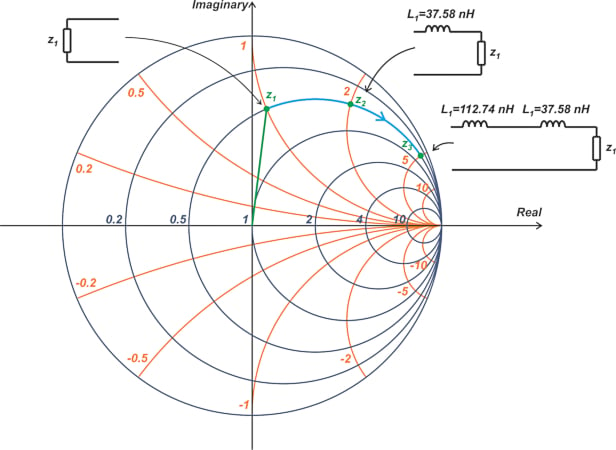##### Figure 5. Smith chart showing the move to z2 = 0.5 + j2.

The figure also shows how adding an additional inductor of 3 × 37.58 nH = 112.74 nH produces an equivalent normalized impedance of 0.5 + j5. As can be seen, adding more and more series inductors moves us along a constant-resistance circle in a clockwise direction. Also, we can say that by increasing the value of an inductor in series with a given impedance, the overall impedance moves along the constant-resistance circle in a clockwise direction.

#### Example 4: A Series RC Network Frequency Sweep

Let’s see how the impedance of a series RC circuit changes with frequency. Assume that R = 25 Ω, C = 10 pF, and Z= 50 Ω. The impedance of a series RC circuit is:

$\begin{eqnarray} Z &=& R+\frac{1}{j \omega C} \\ &=& R-\frac{1}{\omega C}j \end{eqnarray}$

The real part of the normalized impedance works out to r = 0.5. Therefore, the impedance of this circuit is on the constant-resistance circle of r = 0.5. The reactive component of the normalized impedance is $$x = -\frac{1}{\omega CZ_0}$$, which is always negative. As the frequency is swept from DC to infinity, x changes from -∞ to 0. Therefore, regardless of the value of the capacitor, the impedance locus includes the lower half of the r = 0.5 constant-resistance circle.

Note that since x changes from -∞ to 0 with frequency, we move clockwise along the circle as frequency increases. The cyan curve in the following curve (Figure 6) shows the impedance locus on the Smith chart.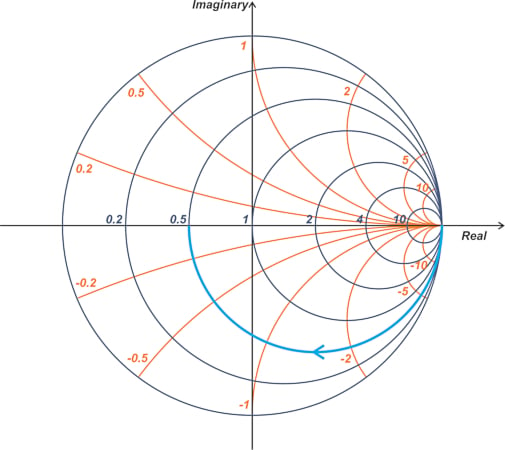#### Example 5: A Series RL Network Frequency Sweep

What would be the impedance contour of a series RL circuit with R = 50 Ω and L = 30 nH if we sweep the frequency from DC to infinity?

With Z= 50 Ω, the impedance of this RL network is on the constant-resistance circle of r = 1. The reactive component of the normalized impedance is $$x=\frac{L \omega}{Z_0}$$, which is always positive. As the frequency is swept from DC to infinity, x changes from 0 to +∞. Therefore, regardless of the value of the inductor, the impedance locus includes the upper half of the r = 1 constant-resistance circle, as shown below in Figure 7.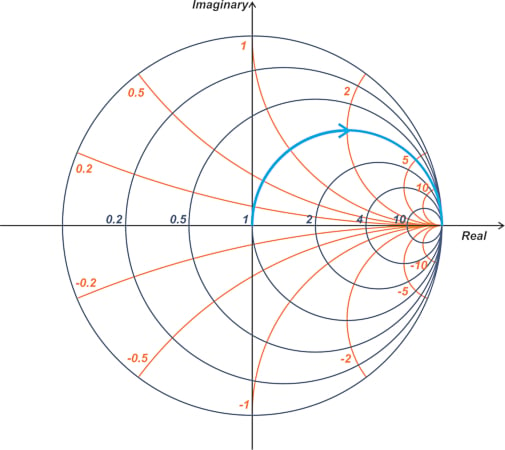##### Figure 7. Smith chart showing the impedance locus on the upper half of r = 1 constant-resistance circuit.

Note that since x changes from 0 to +∞ with frequency, we move clockwise along the circle as frequency increases.

#### Example 6: A Series RLC Network Frequency Sweep

What would be the impedance contour of a series RLC circuit with R = 10 Ω and L = 20 nH, and C = 2 pF if we sweep the frequency from DC to infinity?

With Z= 50 Ω, the impedance of this series RLC network is on the constant-resistance circle of r = 0.2. At the resonant frequency fr, the reactance of the inductor cancels that of the capacitor, leaving us with a purely resistive impedance. Therefore, at fr, we are at the intersection of the r = 0.2 constant-resistance circle and the horizontal diagonal of the Smith chart, as shown below in Figure 8.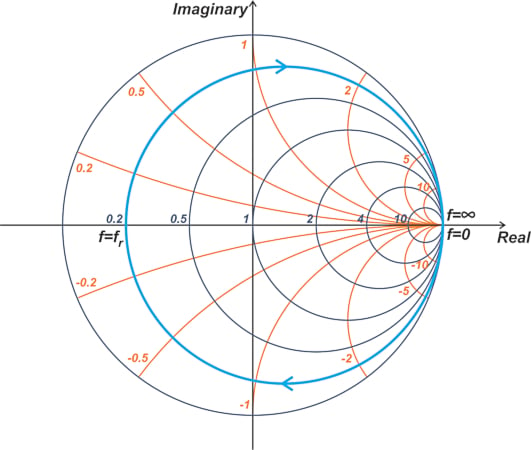##### Figure 8. Smith chart showing fr at the intersection of r = 0.2 constant-resistance circulate and horizontal diagonal.

Below fr, the magnitude of the capacitive reactance is larger than the inductive reactance, and hence the circuit is capacitive. This means that for 0 < f < fr, the circuit is inductive, and we are at the upper half of the constant-resistance circle. On the other hand, for f > fr, the circuit is inductive, and we are at the upper half of the constant-resistance circle. The above examples show that as frequency increases, the impedance contour always follows the constant-resistance circle in a clockwise direction.

### Series Connected Components and the Smith Chart

A series connection of impedances can be most conveniently handled using the impedance Smith chart. Series connected components correspond to a point on the Smith chart. If we sweep the frequency, the imaginary part of the impedance changes. In this case, an impedance contour can be plotted on the Smith chart to describe the circuit behavior over the swept frequency range. It is important to be familiar enough with the Smith chart to recognize what type of circuit configuration can produce a given impedance contour.